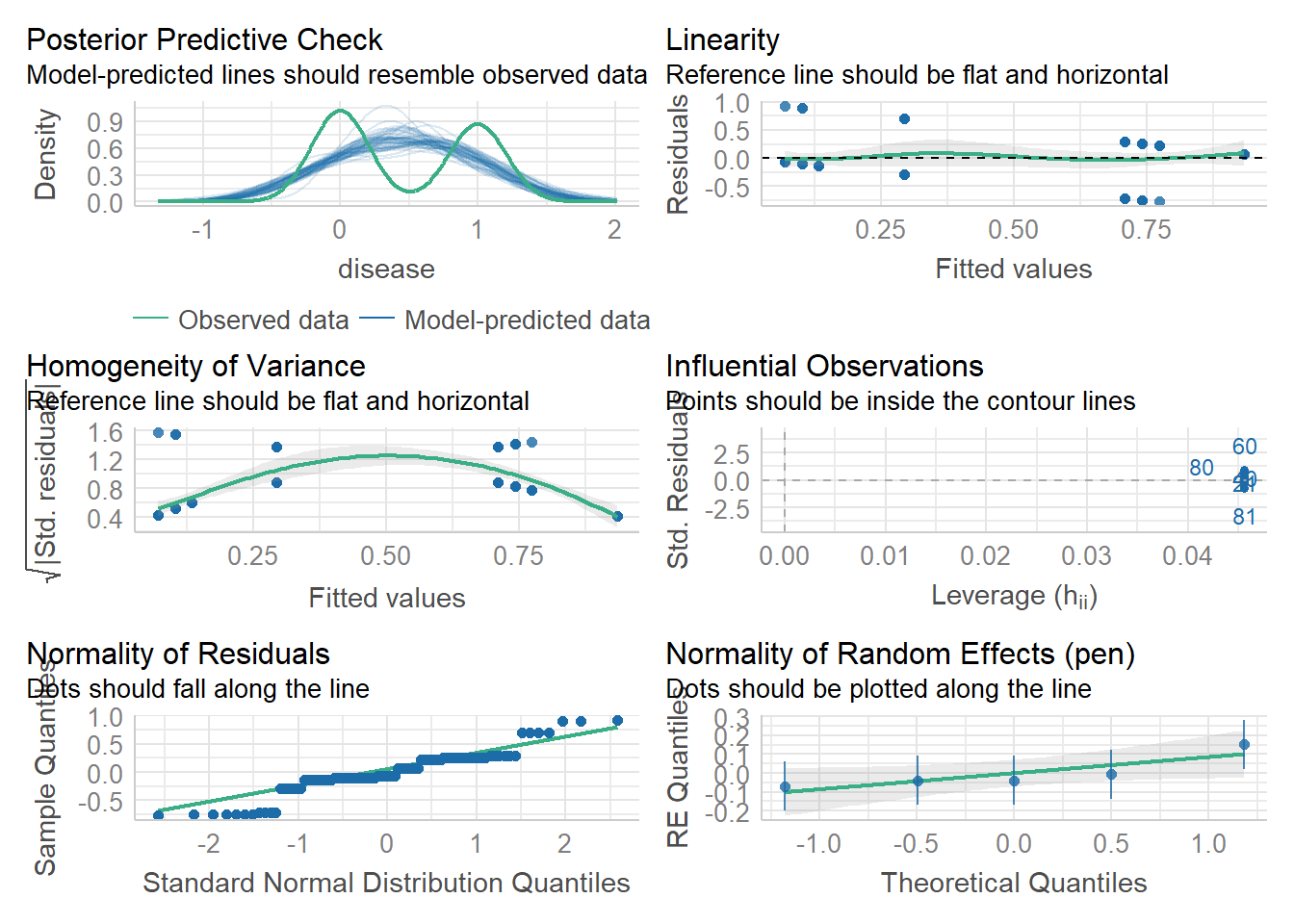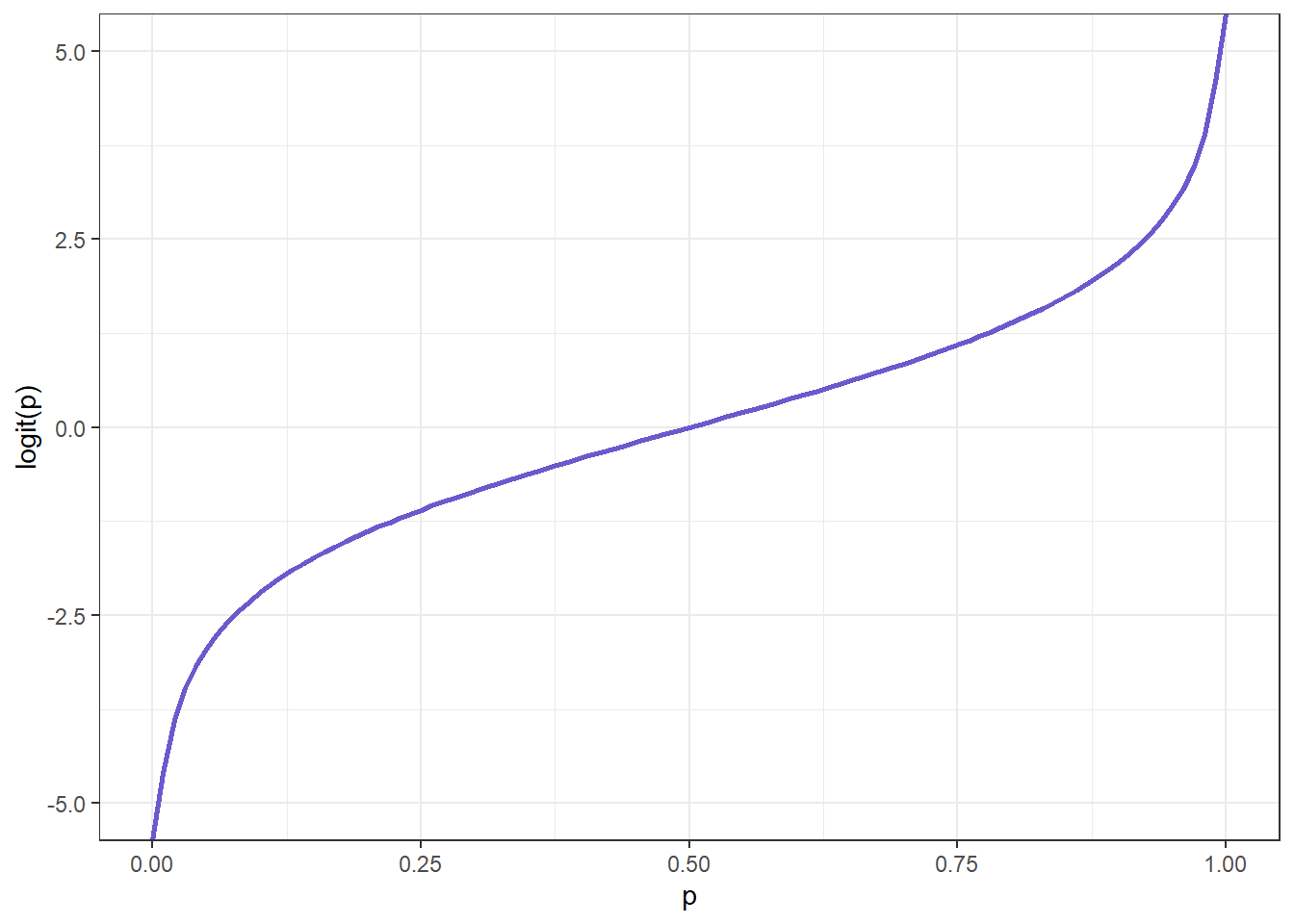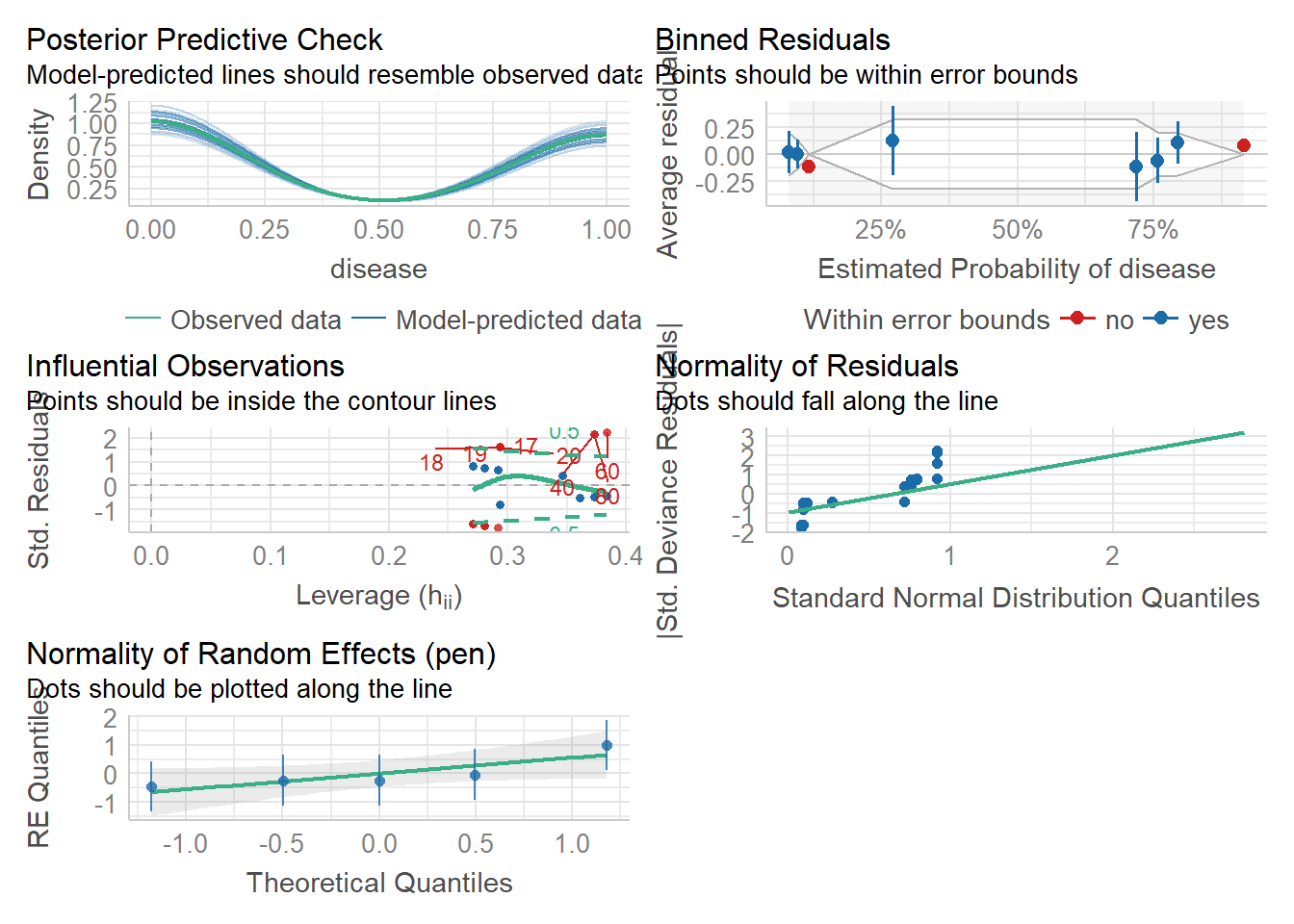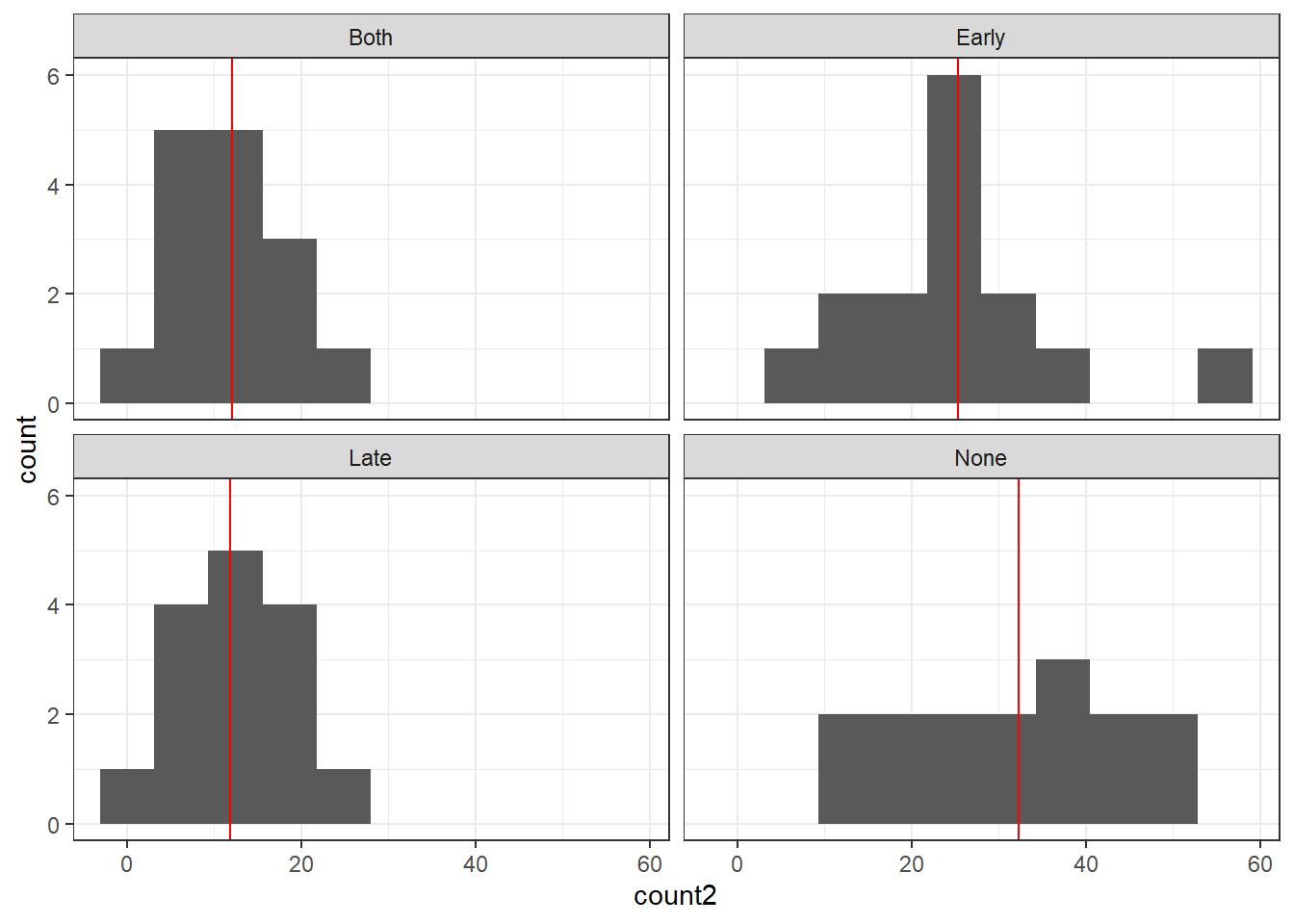# Lesson 5 learning objectives

At the end of this lesson, students will …

• Know what a generalized linear mixed model is and why you might want to use one.
• Fit a generalized linear mixed model to binary outcome data.
• Fit a generalized linear mixed model to count outcome data.

# GLMM with binary response variable

Taking the log transformation of the response variable before fitting the model is a good solution for many types of data. However it won’t always work. For many classes of data, we can’t just do a transformation to “shoehorn” the data into a form that will work with a general linear mixed model. So that means we need to take the next step and enter the world of generalized linear mixed models, or GLMM as they are affectionately known. (It’s a terrible name because general linear mixed model and generalized linear mixed model begin with the same letters, so it’s a little bit confusing, but we are stuck with that.)

Let’s say we have response data that is binary. For example, what if we are testing the ability of a vaccine to prevent some disease in animals? We would have a control group that was not inoculated and a treatment group that was inoculated. The response variable is whether or not the disease is present. The animals are raised in different pens of 10 animals each in a complete block design so we need to account for the effect of pen on disease as well, using a random intercept.

Here is an example of what data from that study might look like.

library(tidyverse)
library(lme4)
library(easystats)
disease <- read_csv('https://usda-ree-ars.github.io/SEAStats/mixed_models_in_R/datasets/disease.csv')
## Rows: 100 Columns: 4
## ── Column specification ────────────────────────────────────────────────────────
## Delimiter: ","
## chr (2): pen, inoc
## dbl (2): animal, disease
##
## ℹ Use spec() to retrieve the full column specification for this data.
## ℹ Specify the column types or set show_col_types = FALSE to quiet this message.

Here I will use the group_by() and summarize() functions to make a table of how many animals in each pen and each treatment got the disease.

disease %>%
group_by(inoc, pen) %>%
summarize(no_disease = sum(disease == 0), disease = sum(disease == 1))
## summarise() has grouped output by 'inoc'. You can override using the
## .groups argument.
## # A tibble: 10 × 4
## # Groups:   inoc 
##    inoc  pen   no_disease disease
##    <chr> <chr>      <int>   <int>
##  1 no    A              0      10
##  2 no    B              3       7
##  3 no    C              4       6
##  4 no    D              3       7
##  5 no    E              1       9
##  6 yes   A              6       4
##  7 yes   B              9       1
##  8 yes   C              9       1
##  9 yes   D              9       1
## 10 yes   E             10       0

What if we tried to just use a linear mixed model as we have done before? We could code disease absent as 0 and disease present as 1.

lmm_disease <- lmer(disease ~ inoc + (1|pen), data = disease)

That seems reasonable at first glance. The intercept is 0.78, so the baseline rate is 78% disease, and the coefficient on inoculation is -0.64, so the inoculation treatment makes disease go down by 64%. What is wrong with that?

Well, what about if we wanted to make predictions? Let’s say we had a population where the baseline rate of disease was only 40%. Our model would predict that it would have -20% disease if we gave that population the inoculation treatment. That is logically impossible. It makes more sense to think about the effect of inoculation in terms of relative changes to the odds of getting the disease. So if the baseline rate is very low, there isn’t that much that inoculation can do to reduce it further. But if the baseline rate is high, we can easily lower it with even a moderately effective treatment.

Furthermore, the residuals of this model will probably not conform to the assumptions of normal distribution and homogeneous variance.

check_model(lmm_disease)
## Not enough model terms in the conditional part of the model to check for
##   multicollinearity.Also take a look at the posterior predictive check diagnostic plot at top left. The predicted values of the model go well outside the 0 to 1 range, leading to a very poor match between the data (green line) and predictions (blue lines).

So what do we do about this? Modeling a binary response can be done with a generalized linear model. We use a link function to convert the predicted response value into a scale that can be normally distributed. What we are predicting is the probability that an individual from each pen with or without the inoculation treatment will get the disease. Probability ranges from 0 to 1, so it cannot really have normally distributed error because normal distributions (bell curves) can take any value, positive or negative. So a bounded range like 0 to 1 doesn’t really play well with normal distributions.

The formula for predicting the probability of disease for individual $$i$$ in pen $$j$$ is as follows:

$\text{logit}~\hat{y}_{i,j} = \beta_0 + \beta_1 inoc_i + u_j$

In this formula, $$\hat{y}_{i,j}$$ is the predicted probability of disease for individual $$i$$ in pen $$j$$. $$\beta_0$$ is the intercept, $$\beta_1$$ is the treatment coefficient, $$inoc_i$$ is a binary variable that is 0 for uninoculated control and 1 for inoculated treatment, and $$u_j$$ is the random effect, a separate intercept for each pen.

But what is that “logit” thing? That is the link function. It is used to transform the probability, which ranges from 0 to 1 and therefore can’t really have normally distributed error, to a scale that can take any value, positive or negative. Logit is another word for the log-odds function, defined as

$\text{logit}~p = \log \frac {p}{1-p}$

Here is a graph of that function:You can see that it maps the values ranging from 0 to 1 to values ranging from negative to positive infinity. It is more or less a straight line when the probability is between about 0.25 and 0.75, but starts to get really steep as you get close to the boundaries. This makes sense because if you have an event that has an extremely low (or high) chance of happening, a little increase (or decrease) to the probability will be a relatively bigger difference.

So to use statistical jargon, what we are going to do here is fit a binomial GLMM (binomial meaning our response variable is a binary outcome) with a logit link function to transform the predicted probabilities onto the log odds scale.

We use the glmer() function instead of lmer() in this case. (If this was SAS, we would be using proc glimmix.) We specify a formula in the same way as we have been doing with lmer(), and the data frame from which the variables come, but we now have a new argument, family. This refers to the “family” of response distributions that we are going to be using. Here we specify binomial(link = 'logit'). Other link functions are possible to use with binomial GLMMs, but we will not get into that today.

glmm_disease <- glmer(disease ~ inoc + (1|pen), data = disease, family = binomial(link = 'logit'))

What do the diagnostic plots look like now?

check_model(glmm_disease)
## Not enough model terms in the conditional part of the model to check for
##   multicollinearity.This looks much better especially the posterior predictive check. All model predictions are between 0 and 1 which makes logical sense because they are probabilities, and also is a very good match to the observations. The residuals look better as well.

What does this model tell us?

summary(glmm_disease)
## Generalized linear mixed model fit by maximum likelihood (Laplace
##   Approximation) [glmerMod]
##  Family: binomial  ( logit )
## Formula: disease ~ inoc + (1 | pen)
##    Data: disease
##
##      AIC      BIC   logLik deviance df.resid
##     97.2    105.0    -45.6     91.2       97
##
## Scaled residuals:
##     Min      1Q  Median      3Q     Max
## -1.9600 -0.3627 -0.2951  0.5659  3.3881
##
## Random effects:
##  Groups Name        Variance Std.Dev.
##  pen    (Intercept) 0.4676   0.6838
## Number of obs: 100, groups:  pen, 5
##
## Fixed effects:
##             Estimate Std. Error z value Pr(>|z|)
## (Intercept)   1.3914     0.4826   2.883  0.00394 **
## inocyes      -3.3741     0.6244  -5.404 6.53e-08 ***
## ---
## Signif. codes:  0 '***' 0.001 '**' 0.01 '*' 0.05 '.' 0.1 ' ' 1
##
## Correlation of Fixed Effects:
##         (Intr)
## inocyes -0.534

Now we have an intercept of 1.39 and a coefficient on the inoculation treatment of -3.37. Great, but what does that exactly mean? When we are dealing with models that have link functions, the coefficients are hard to interpret at first glance because they are on the log odds scale. We have to back-transform them to the probability scale to make any sense of them.

The intercept of 1.39 is the fitted log odds of getting the disease in the uninoculated control, averaged across the random effect of pen. To transform this log odds back to the probability scale ranging from 0 to 1, we use the inverse logit function, plogis().

plogis(1.39)
##  0.8005922

So the model population-level prediction is that a random individual who isn’t inoculated has 80% probability of getting the disease.

What about the slope of -3.37? That is the change in the log odds between uninoculated and inoculated. To find out the model prediction for inoculated individuals, we need to subtract 3.37 from the intercept, then take the inverse logit of that value.

plogis(1.39 - 3.37)
##  0.1213188

The probability of getting the disease, says the model, plummets from 80% down to 12% if the individual is inoculated.

In the next lesson, we will look at easier ways to extract model predictions from GLMM models – you will not have to do it “by hand” as we just did.

We can set up a generalized linear mixed model with all the same kinds of fixed and random effects as in plain vanilla linear mixed models. For example, we can have continuous and categorical predictors, interactions, crossed or nested random effects, random intercepts and/or random slopes. I will leave that for the exercises.

# GLMM with count response variable

If your response variable is a discrete count, you have a similar issue as with binary response data. Your response variable can only be a non-negative integer, 0 or greater. It’s easier to imagine a “bell curve” looking distribution for count data. But we still have the issue that there are only discrete values, not continuous as we would have to have for a true normal distribution. Also, we can easily end up with a model that predicts negative counts, which is basically nonsense.

GLMMs come to the rescue again! In this case, we will use a Poisson distribution with a log link function. The Poisson distribution is bounded over non-negative integers, so it is ideal for count data (as long as there are not too many 0 values).

Here we are going to use an example dataset from the agridat package, like we did for the exercises in the previous lesson. This dataset comes from an experiment conducted by George Stirret and colleagues in Canada in 1935 to determine whether fungal spores could be applied to corn plants to control the European corn borer. There were four levels of the fungal spore treatment (trt column in the dataset): an untreated control ("None"), early fungal treatment ("Early"), late fungal treatment ("Late"), and a treatment in which fungal spores were applied both early and late ("Both"). There were 15 experimental blocks (block column), each containing four plots. The number of borers per plot was counted on two different dates. For simplicity we will only consider the second count (count2 column).

data('stirret.borers', package = 'agridat')

If you are running R locally and for some reason you do not have access to the agridat package, I have provided the data in a CSV file:

stirret.borers <- read_csv('https://usda-ree-ars.github.io/SEAStats/mixed_models_in_R/datasets/stirret.borers.csv')

Here is a histogram of the count2 data for each treatment separately, set to have 10 bins using the geom_histogram() function from ggplot2, and the facet_wrap() function to make a separate panel for each treatment. I’ve also included a vertical line at the mean for each treatment.

borer_means <- stirret.borers %>%
group_by(trt) %>%
summarize(count2_mean = mean(count2))

ggplot(stirret.borers, aes(x = count2)) +
geom_histogram(bins = 10) +
geom_vline(aes(xintercept = count2_mean), data = borer_means, color = 'red') +
facet_wrap(~ trt) +
theme_bw()You can see that we have quite a few counts of zero or close to it, and that the “Both” and “Late” treatments have the lowest mean counts, with “Early” higher and “None” the highest.

As we’ve been doing, let’s rearrange the factor levels of the trt column so that the control level ’None is first in the ordering and will be treated as the reference level by the model. This will assist our interpretation.

stirret.borers <- stirret.borers %>%
mutate(trt = factor(trt, levels = c('None', 'Early', 'Late', 'Both')))

For a proof of concept, let’s once again fit the model as a general linear model (with random intercepts), assuming normally distributed residuals.

lmm_borers <- lmer(count2 ~ trt + (1|block), data = stirret.borers)

Then fit the generalized linear model. Here we use family = poisson(link = 'log') as the link function that works best with the Poisson distribution is just log().

glmm_borers <- glmer(count2 ~ trt + (1|block), data = stirret.borers, family = poisson(link = 'log'))

Compare the diagnostic plots from each model, first from the linear mixed model:

check_model(lmm_borers)
## Not enough model terms in the conditional part of the model to check for
##   multicollinearity.`